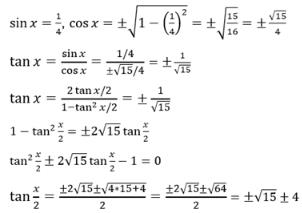Courses

# Test: Multiple Angle Formula

## 10 Questions MCQ Test Mathematics (Maths) Class 11 | Test: Multiple Angle Formula

Description
This mock test of Test: Multiple Angle Formula for JEE helps you for every JEE entrance exam. This contains 10 Multiple Choice Questions for JEE Test: Multiple Angle Formula (mcq) to study with solutions a complete question bank. The solved questions answers in this Test: Multiple Angle Formula quiz give you a good mix of easy questions and tough questions. JEE students definitely take this Test: Multiple Angle Formula exercise for a better result in the exam. You can find other Test: Multiple Angle Formula extra questions, long questions & short questions for JEE on EduRev as well by searching above.
QUESTION: 1

### Find the value of  sin θ/3

Solution: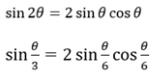QUESTION: 2

### tan 2x =

Solution: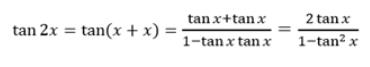QUESTION: 3

### What is the value of tan 3θ ? if tan θ = 1/2

Solution: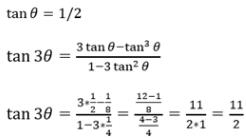QUESTION: 4

If cos θ = 1/√5 what is the value of cos 2θ?

Solution: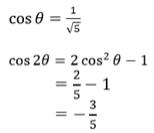QUESTION: 5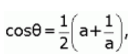then the value of cos 2θ is

Solution: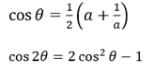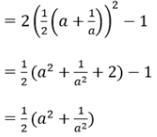QUESTION: 6

Find tan θ/3

Solution:
QUESTION: 7

The value of tan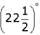Solution: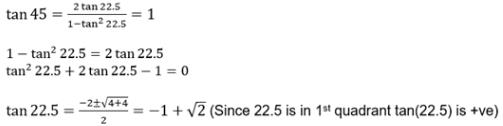QUESTION: 8

When sinθ + cosθ = 1, then the value of sin2θ is

Solution:

Squaring on both sides,you get sin2x + 2sinxcosx + cos2x = 1

which gives 1 + 2sinxcosx = 1 {since sin2x +cos2x = 1 for all x}

which means,2sinxcosx =0

which means either sinx =0 or cosx=0

so,the general solution is that for sinx=0 union cosx=0

QUESTION: 9

What is the value of cosθ/2 ?

Solution:
QUESTION: 10

sin x = 1/4 ; the possible values of tan x/2 are

Solution: